Indexing and Referencing

NHDPlus Flowline Indexing

First we’ll load up some data. In this case, we use flowlines from the NHDPlus subset that’s included in the package and a set of points to index. We’ll use the NHDPlus Gages layer for this example. The data in this example is big. The R session needs a lot of memory to hold the whole NHDPlus flowline layer and run the calculations.

library(nhdplusTools)

nhdplus_path(file.path(work_dir, "natseamless.gpkg"))

Now we can call nhdplusTools::get_flowline_index() on the data we just loaded. Note that we are doing our spatial searching in units of degrees. The get_flowline_index has an input, search_radius which defaults to 0.1. See the documentation of the nn2 function from the RANN package for more information on how the search works.

NOTE: If you have a small area in which you need flowline indexes, get_flowline_index() has an option to download flowlines in the bounding box of your input points.

indexes <- get_flowline_index(flowlines,
sf::st_geometry(gages),
max_matches = 1)

indexes <- left_join(sf::st_sf(id = c(1:nrow(gages)),
geom = sf::st_geometry(gages)),
indexes, by = "id")

plot(sf::st_geometry(sf::st_zm(flowlines)))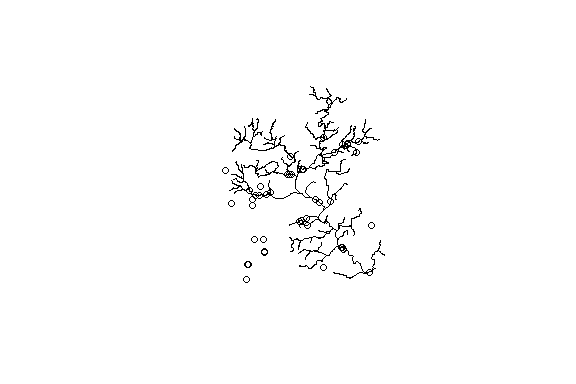Now let’s look at the results and see how the nhdplusTools::get_flowline_index() did. The below shows the percent of COMIDs and REACHCODEs that match and shows a histogram of the measure differences for the REACHCODEs that were matched.

p_match <- 100 * length(which(indexes$COMID %in% gages$FLComID)) / nrow(gages)
paste0(round(p_match, digits = 1),
"% were found to match the COMID in the NHDPlus gages layer")
#>  "63% were found to match the COMID in the NHDPlus gages layer"

p_match <- 100 * length(which(indexes$REACHCODE %in% gages$REACHCODE)) / nrow(gages)
paste0(round(p_match, digits = 1),
"% were found to match the REACHCODE in the NHDPlus gages layer")
#>  "65.2% were found to match the REACHCODE in the NHDPlus gages layer"

matched <- cbind(indexes,
dplyr::select(sf::st_drop_geometry(gages),
REACHCODE_ref = REACHCODE,
COMID_ref = FLComID,
REACH_meas_ref = Measure)) %>%
dplyr::filter(REACHCODE == REACHCODE_ref) %>%
dplyr::mutate(REACH_meas_diff = REACH_meas - REACH_meas_ref)

hist(matched$REACH_meas_diff, breaks = 100, main = "Difference in measure for gages matched to the same reach.")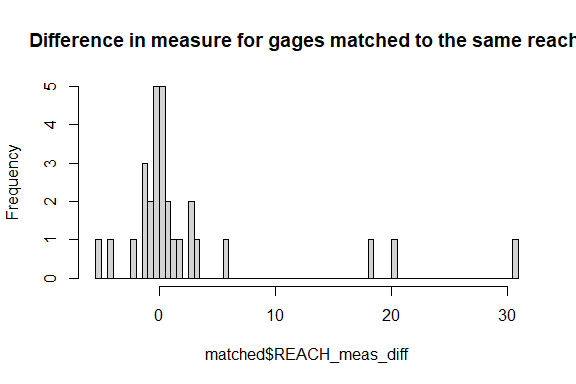round(quantile(matched$REACH_meas_diff,
probs = c(0, 0.1, 0.25, 0.5, 0.75, 0.9, 1)),
digits = 2)
#>    0%   10%   25%   50%   75%   90%  100%
#> -5.11 -1.40 -0.52  0.36  1.57  8.11 30.74

Flowline Indexing with higher precision

The above example used the native nodes of the NHDPlus as the potential measure snap locations. The nhdplusTools::get_flowline_index() function has the ability to refine these by segmentizing the line to some given resolution. Let’s try the same thing using a resolution of 10m and see if we can do any better.

Note that the sf::st_segmentize function takes care of the distance conversion and segmentizes our lon/lat lines to 10m on the fly.

indexes <- get_flowline_index(flowlines,
sf::st_geometry(gages),
precision = 10)

indexes <- left_join(data.frame(id = seq_len(nrow(gages))), indexes, by = "id")

Now lets look at out comparison again.

p_match <- 100 * length(which(indexes$COMID %in% gages$FLComID)) / nrow(gages)
paste0(round(p_match, digits = 1),
"% were found to match the COMID in the NHDPlus gages layer")
#>  "69.6% were found to match the COMID in the NHDPlus gages layer"

p_match <- 100 * length(which(indexes$REACHCODE %in% gages$REACHCODE)) / nrow(gages)
paste0(round(p_match, digits = 1),
"% were found to match the REACHCODE in the NHDPlus gages layer")
#>  "71.7% were found to match the REACHCODE in the NHDPlus gages layer"

matched <- cbind(indexes,
dplyr::select(sf::st_set_geometry(gages, NULL),
REACHCODE_ref = REACHCODE,
COMID_ref = FLComID,
REACH_meas_ref = Measure)) %>%
dplyr::filter(REACHCODE == REACHCODE_ref) %>%
dplyr::mutate(REACH_meas_diff = REACH_meas - REACH_meas_ref)

hist(matched$REACH_meas_diff, breaks = 100, main = "Difference in measure for gages matched to the same reach.")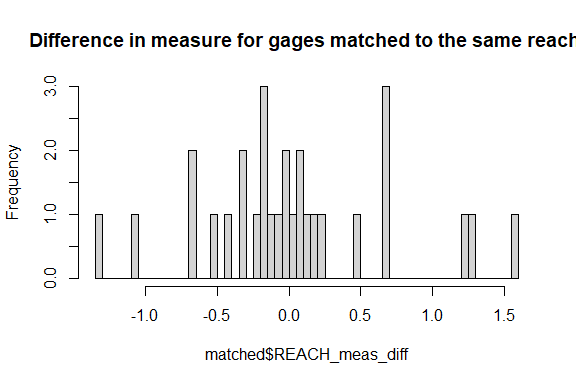round(quantile(matched$REACH_meas_diff,
probs = c(0, 0.1, 0.25, 0.5, 0.75, 0.9, 1)), digits = 2)
#>    0%   10%   25%   50%   75%   90%  100%
#> -1.31 -0.66 -0.33 -0.04  0.22  0.81  1.57

Finding multiple indexes

get_flowline_index() has a parameter max_matches that controls how many indexed flowlines are returned per point. This is useful for points that are near many flowlines and some further disambiguation is needed to determine exactly which flowline the point should be indexed to. At the time of writing, that functionality is not included in nhdplusTools but is planned.

For this example, we’ll just look at one point.

indexes <- get_flowline_index(flowlines,
sf::st_geometry(gages),
max_matches = 10)

indexes <- left_join(sf::st_sf(id = 1,
geom = sf::st_geometry(gages)),
indexes, by = "id")

plot(sf::st_geometry(sf::st_buffer(indexes, 0.005)), border = NA)
plot(sf::st_geometry(sf::st_zm(flowlines)), col = "blue", add = TRUE)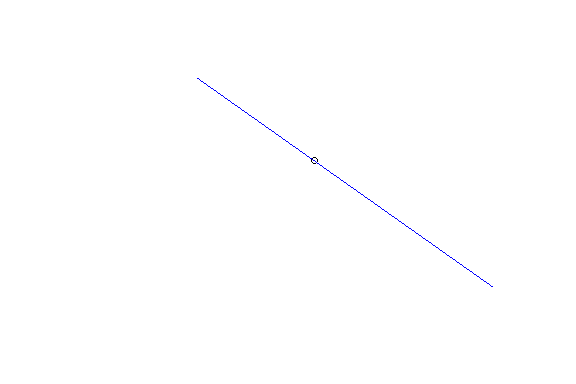indexes
#> Simple feature collection with 10 features and 5 fields
#> Geometry type: POINT
#> Dimension:     XY
#> Bounding box:  xmin: -89.35278 ymin: 43.20867 xmax: -89.35278 ymax: 43.20867
#> Geodetic CRS:  GRS 1980(IUGG, 1980)
#>    id    COMID      REACHCODE REACH_meas       offset
#> 1   1 13293452 07090002007737     0.0000 0.0004868953
#> 2   1 13293456 07090002007738   100.0000 0.0004868953
#> 3   1 13293432 07090002007736   100.0000 0.0004868953
#> 4   1 13293430 07090002007639     0.0000 0.0008846702
#> 5   1 13293454 07090002007638   100.0000 0.0008846702
#> 6   1 13294394 07090002007637   100.0000 0.0049572540
#> 7   1 13294128 07090002007636   100.0000 0.0052999159
#> 8   1 13294382 07090002007725     0.0000 0.0052999159
#> 9   1 13294274 07090002007725     3.6313 0.0065388475
#> 10  1 13293458 07090002007725    12.5173 0.0099172984
#>                          geom
#> 1  POINT (-89.35278 43.20867)
#> 2  POINT (-89.35278 43.20867)
#> 3  POINT (-89.35278 43.20867)
#> 4  POINT (-89.35278 43.20867)
#> 5  POINT (-89.35278 43.20867)
#> 6  POINT (-89.35278 43.20867)
#> 7  POINT (-89.35278 43.20867)
#> 8  POINT (-89.35278 43.20867)
#> 9  POINT (-89.35278 43.20867)
#> 10 POINT (-89.35278 43.20867)

Waterbody Indexing

The get_flowline_index() function estimates a hydrographic address as a linear reference to a flowline. For points near bodies of water, these could be an inappropriate kind of index. This is because where flowlines run through a waterbody. they are “artificial paths” and do not represent the waterbody. The get_waterbody_index() function is intended to address points that are in or near the shore of a waterbody.

This next block of code loads the NHDPlus Waterbody layer and creates an interactive map. Of interest on gages that are near the short of bodies of water but far away from flowlines. Note that we drop the NHDPlus geometry and use the source LonSite and LatSite attributes for geometry.

gages <- sf::st_drop_geometry(gages) %>%
dplyr::filter(!is.na(LonSite)) %>%
sf::st_as_sf(coords = c("LonSite", "LatSite"), crs = 4326)

plot(sf::st_geometry(sf::st_zm(flowlines)))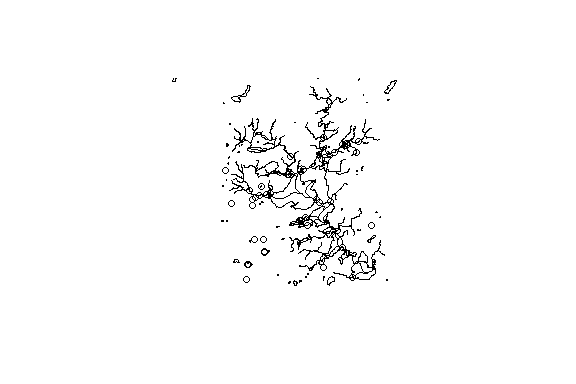This next block shows how to call get_flowline_index() and get_waterbody_index() and what the output looks like.

flowline_indexes <- left_join(data.frame(id = seq_len(nrow(gages))),
get_flowline_index(
sf::st_transform(flowlines, 5070),
sf::st_geometry(sf::st_transform(gages, 5070)),
search_radius = 200), by = "id")

indexed_gages <- cbind(dplyr::select(gages,
orig_REACHCODE = REACHCODE,
orig_Measure = Measure,
FLComID,
STATION_NM),
flowline_indexes,
get_waterbody_index(
st_transform(waterbody, 5070),
st_transform(gages, 5070),
st_drop_geometry(flowlines),

plot(sf::st_geometry(sf::st_zm(flowlines)))dplyr::select(sf::st_drop_geometry(indexed_gages), near_wb_COMID, near_wb_dist, in_wb_COMID, outlet_fline_COMID)
#>    near_wb_COMID near_wb_dist in_wb_COMID outlet_fline_COMID
#> 1             NA           NA          NA                 NA
#> 2       13293226    11.013063          NA           13294384
#> 3             NA           NA          NA                 NA
#> 4      167120949    16.862351          NA           13294360
#> 5             NA           NA          NA                 NA
#> 6             NA           NA          NA                 NA
#> 7       13293262    22.640184          NA           13294312
#> 8             NA           NA          NA                 NA
#> 9       13296360    50.380329          NA           13297172
#> 10            NA           NA          NA                 NA
#> 11            NA           NA          NA                 NA
#> 12      13293262    64.925912          NA           13294312
#> 13      13293262   164.160184          NA           13294312
#> 14     167120949    39.327388          NA           13294360
#> 15            NA           NA          NA                 NA
#> 16            NA           NA          NA                 NA
#> 17            NA           NA          NA                 NA
#> 18      13293284    57.456159    13293284                 NA
#> 19      13293262    33.292260          NA           13294312
#> 20            NA           NA          NA                 NA
#> 21            NA           NA          NA                 NA
#> 22            NA           NA          NA                 NA
#> 23      13293226   181.370856          NA           13294384
#> 24            NA           NA          NA                 NA
#> 25      14711422    22.566279          NA                 NA
#> 26            NA           NA          NA                 NA
#> 27            NA           NA          NA                 NA
#> 28            NA           NA          NA                 NA
#> 29            NA           NA          NA                 NA
#> 30            NA           NA          NA                 NA
#> 31            NA           NA          NA                 NA
#> 32            NA           NA          NA                 NA
#> 33            NA           NA          NA                 NA
#> 34            NA           NA          NA                 NA
#> 35            NA           NA          NA                 NA
#> 36      13293316    37.633591    13293316           13294374
#> 37            NA           NA          NA                 NA
#> 38            NA           NA          NA                 NA
#> 39      13293322     6.737635          NA           13294344
#> 40            NA           NA          NA                 NA
#> 41            NA           NA          NA                 NA
#> 42      13293322     3.914543          NA           13294344
#> 43            NA           NA          NA                 NA
#> 44      13293322     6.737635          NA           13294344
#> 45      13293262    50.163000          NA           13294312